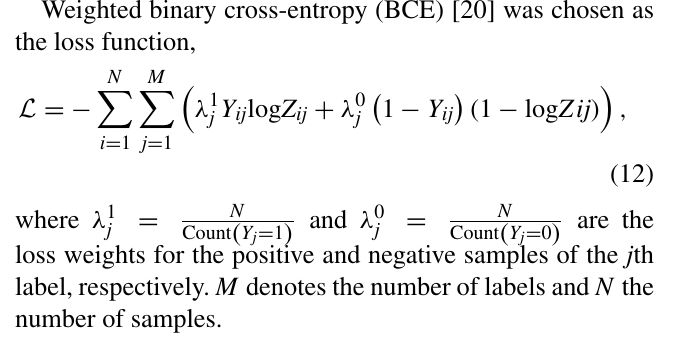# Pos_weight attribute in BCEWithLogitLoss

I am attempting to work on the following loss function. To my understanding, this is essentially a BCE loss function where we need to work with the weight parameter. I initially started with

1. Counting the number of positive examples and then `weight = total_samples / number_positive_per_class`. However, clearly this is not what the paper suggests.

2. I read through the documents and it seems I need to set the `pos_weight` parameter. However, there is nothing to set the `neg_weights` as might be needed in this case. So, can I use the ratio of `pos_weight = neg_samples/ pos_samples`

3. If this should be the way, can I use the direct ratio or I need to do something else in order to ensure the loss values are correctly weighted?Any help would be highly appreciated. Thank you

I think you could transform the posted formula into the `pos_weight` formula used in `nn.BCEWithLogitsLoss` as given in the docs.

As described, `pos_weight` is specified as `nb_neg/nb_pos` and is multiplied with the “positive” part of the loss (left summand). If you divide both summands by `lambda_0` in your formula, you would end up with the same loss formulation.

Thank you so much for the reply. Here is a followup though. I see there is also an implementation for the function `MultiLabelSoftMarginLoss`. I think in spirit it is similar to the `BCEWithLogitLoss` and since I have a multi label classification, I thought it would be better to use the `MultiLabelSoftMarginLoss` function. However, there is no `pos_weight` parameter in it. Is there a way I can use it or should I stick to `BCEWithLogitLoss`.

Also, just to be sure, should I use `pos_weight = neg_samples_of_each_class/ pos_samples_of_each_class` in the loss formulation?

I don’t know how `MultiLabelSoftMarginLoss` would compare to `BCEWithLogitsLoss`, so let’s wait for some experts to chime in.

In case you are dealing with a multi-label classification, `pos_weight` should get a value for each class, i.e. it should be a tensor containing `nb_classes` values defined as `[nb_neg_class0/nb_pos_class0, nb_neg_class1/nb_pos_class1, ...]`.

1 Like

Thank you for the quick response. Just to confirm, even if I use only the `BCEWithLogitLoss`, it should be fine in the Multi-label scenario. I just want to make sure that the loss I am computing in this way, is not incorrect.

Yes, `nn.BCEWithLogitsLoss` can be used for binary and multi-label classification use cases.

1 Like